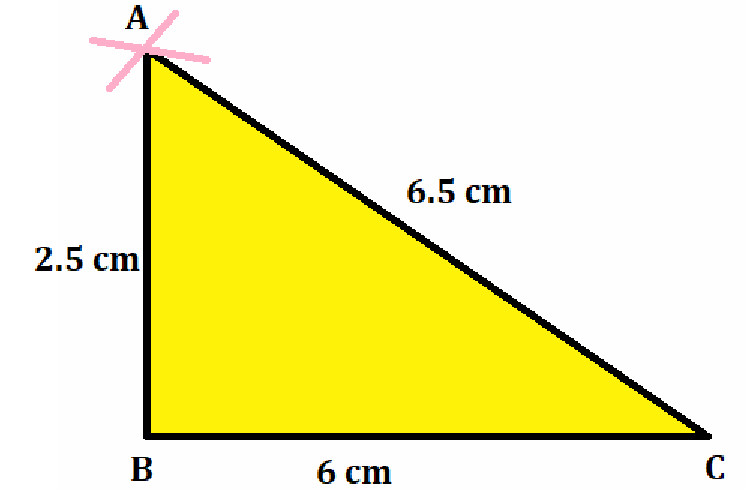# Construct ΔABC such that AB = 2.5 cm, BC = 6 cm and AC = 6.5 cm. Measure ∠B.

Solution>

The steps for construction are given below:

1. Draw a line segment BC = 6 cm.

2. Considering B as a center and radius 2.5 cm, draw an arc.

3. Considering C as a center and radius 6.5 cm, draw another arc, cutting the previous arc at A.

4. Join AB and AC.

Then, ΔABC obtained is the required triangle.

5. When we measure the angle B of triangle by protractor, then angle is equal to ∠B = 90o(0)(0)